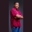Related Tags

string
decimal
c#
communitycreator

# How to convert a string to a decimal in C#Theodore Kelechukwu Onyejiaku

### Overview

Converting a string to a decimal value or decimal equivalent can be done using the Decimal.TryParse() method.

It converts the string representation of a number to its decimal equivalent. When a value is returned by this method, the conversion was successful.

### Syntax

public static bool TryParse (string s, out n);
Syntax

### Parameters

• s: This is the string that we want to convert to a decimal value.

• n: This is a mandatory parameter that is a decimal value. When the Decimal.TryParse() method is called, it returns the decimal value. This value is stored inside n.

### Return value

It returns a Boolean value. It returns true if string s was successfully converted. Otherwise, it returns false.

### Example

// use System
using System;
// create class
class StringToDecimal
{
// main method
static void Main()
{
// create string values
string s1 = "34.43";
string s2 = "hello";
string s3 = "10.34";

// create decimal value that will
// store results of conversions
decimal n1;

// if successful conversion
if(Decimal.TryParse(s1, out n1)){
// print string, result and instance type
Console.WriteLine(
"Value = {0}, result = {1}, type : {2}",
s1, n1, s1.GetType());

}else{
Console.WriteLine("Conversion of {0} failed", s1);
}

// if successful
if(Decimal.TryParse(s2, out n1)){
// print string, result and instance type
Console.WriteLine(
"Value = {0}, result = {1}, type : {2}",
s2, n1, s2.GetType());
}else{
// else
Console.WriteLine("Conversion of {0} failed", s2);
}

// if successful
if(Decimal.TryParse(s3, out n1)){
// print string, result and instance type
Console.WriteLine(
"Value = {0}, result = {1}, type : {2}",
s3, n1, s3.GetType());
}else{
// else
Console.WriteLine("Conversion of {0} failed", s3);
}
}
}
Convert a string to a decimal in C#

### Explanation

• Lines 10–12: We create some string variables.

• Line 16: We create a decimal variable that is not yet initialized with a value. It will store the value converted by the Decimal.TryParse() method.

• Line 19–49: We check if the conversion of string s1, s2, and s3 was successful using if and else statements. If it is successful, then we print the value of the string, the result, and instance type. Otherwise, we say that the conversion failed.

Note: When the code is run, s2 fails to convert because it does not contain a numerical value.

RELATED TAGS

string
decimal
c#
communitycreator

CONTRIBUTORTheodore Kelechukwu Onyejiaku
RELATED COURSES

View all Courses

Keep Exploring

Learn in-demand tech skills in half the time